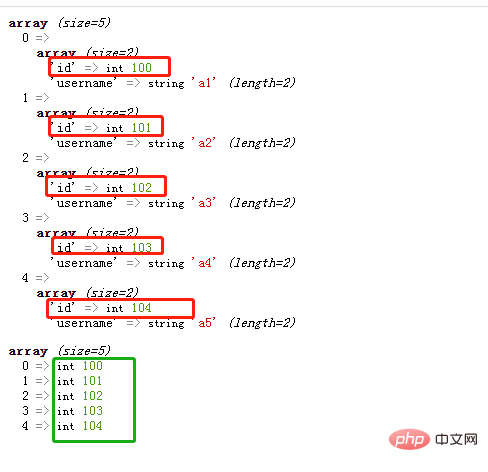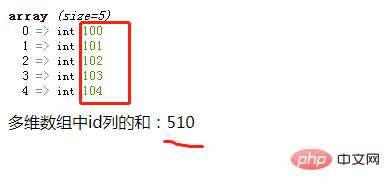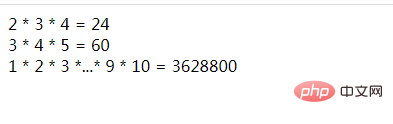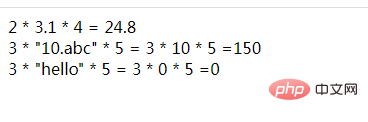# php数组中指定一列求和用什么函数

php数组中指定一列求和用array_column()和array_sum()函数。步骤：1、用array_column()获取数组中指定一列的全部元素，语法“array_column(数组, '指定列名')”，会返回一个包含全部元素的结果数组；2、用array_sum()函数计算结果数组中所有元素的和即可，语法“array_sum(结果数组)”。php数组中指定一列求和用array_column()和array_sum()函数。

• array_column()函数可以获取数组中指定一列的全部元素

• array_sum()函数可计算数组中所有元素的和

array_column() 可以返回指定数组中某个单一列的值；会返回一个数组，数组值为就是指定一列的值。

```<?php
\$user = array(
'0' => array('id' => 100, 'username' => 'a1'),
'1' => array('id' => 101, 'username' => 'a2'),
'2' => array('id' => 102, 'username' => 'a3'),
'3' => array('id' => 103, 'username' => 'a4'),
'4' => array('id' => 104, 'username' => 'a5'),
);
var_dump(\$user);
\$id=array_column(\$user, 'id');
var_dump(\$id);
?>```array_sum() 函数可以计算指定数组中所有元素的和

```\$sum=array_sum(\$id);
echo "多维数组中id列的和：".array_sum(\$id);```array_sum()函数可以计算数组元素总和，而array_product()函数可以计算数组元素乘积。

```<?php
\$array= array(2,3,4);
echo '2 * 3 * 4 = '. array_product(\$array);

\$array= array(3,4,5);
echo '<br>3 * 4 * 5 = '. array_product(\$array);

\$array= array(1,2,3,4,5,6,7,8,9,10);
echo '<br>1 * 2 * 3 *...* 9 * 10 = '. array_product(\$array);
?>``````<?php
\$array= array(2,"3.1",4);
echo '2 * 3.1 * 4 = '. array_product(\$array);

\$array= array(3,"10.abc",5);
echo '<br>3 * "10.abc" * 5 = 3 * 10 * 5 ='. array_product(\$array);

\$array= array(3,"hello",5);
echo '<br>3 * "hello" * 5 = 3 * 0 * 5 ='. array_product(\$array);
?>```
• 字符串"3.1"会被转换成小数 3.1 ，所以第一个数组的元素乘积为24.8。

• 字符串 "10abc" 会被转换成整数 10，所以第二个数组的元素乘积为150。

• 字符串“hello”无法被转换成整数，因此作为 0 值，继而第三个数组的元素乘积为0。#### 相关文章推荐

• php怎么查询类中方法是否存在• php中的时间戳怎么转换成时间• php页面提交方式有什么不同• php怎么获取当前日期和星期几• php怎么将html实体转义为字符
1/1